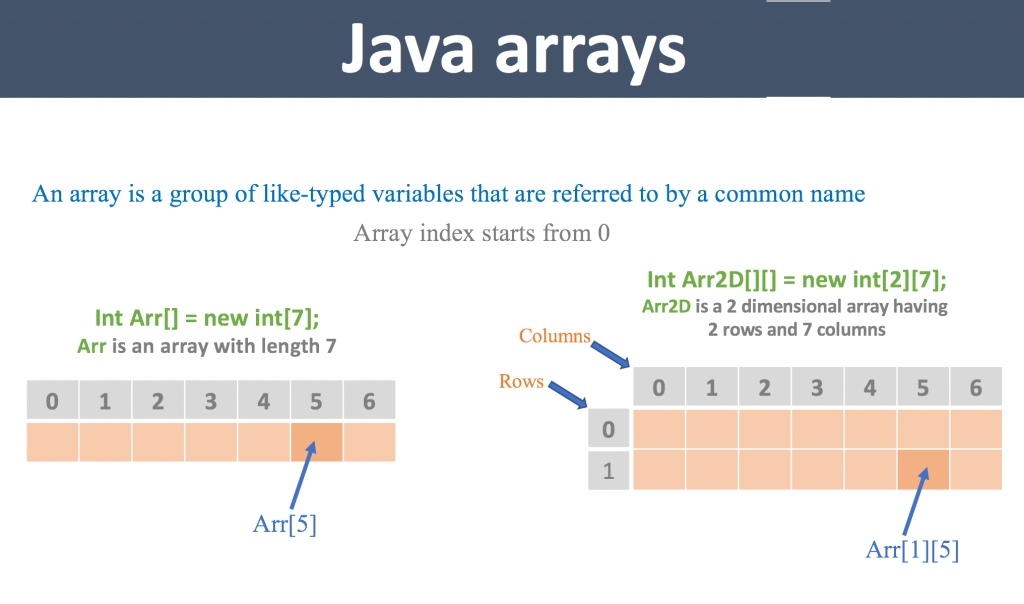JavaForQA#### What is Array

• Arrays are used to store homogenous elements/values (of same data type)
• Can access the elements by the indexes.
• Index always start from zero (0).
• Arrays can be single or multi dimensional

#### Watch demo –

Let’s see some of the implementation of the array.#### One dimensional array:

dataType[] varName  //declaring array variable
varName = new dataTyp[Size of array]
e.g: int a[]; or int[] a;
a = new int;

```/*String[] names = new String; //A total of 3 elements can hold
*names = "sun";
*names = "moon";
*names = "mars";
*/
//or directly we can declare and assign values
String[] names = {"sun", "moon", "mars"}; //index starts from 0
system.out.println("No  of elements:"+names.length); //0 to name.length-1
for(int i=0;i<names.length;i++)
{
system.out.println(names[i]); //prints each element of array
}
//Another type of for loop
for(String name: names)
{
system.out.println(name);
}
```

So we have 2 ways to declare and assign values:

• Declare the array variable and specify the size, and then assign values to each index
• Declare and assign values then n there, no need to specify the size.

#### Two dimensional array:

Let’s see different ways of declaring, assigning and accessing 2 dimensional array in java –
```int arr2d[][] = new int;
//or, we can declare each row size
/*int arr2d[][] = new int[]; //atleast u must specify the size of 1st dimension, Row Size
*int arr2d = new int;
*int arr2d = new int;
*int arr2d = new int; */
//Assign values
arr2d = 1;
arr2d = 2;
arr2d = 3;
arr2d = 4;
arr2d = 5;
arr2d = 6;
//or, declare and assign values, no need to specify size
int[][] arr2d = {{1,2,3},{4,5,6}};
//system.out.println("Rows:"+arr2d.length); //Output: Rows:2
//system.out.println("Columns on 1st Row:"arr2d.length); //Output:Columns on 1st Row:3
//Get the 2d array values using 2 loops
for (int i = 0; i < arr2d.length; i++)
{
for (int j = 0; j < arr2d[i].length; j++)
{
system.out.print(arr2d[i][j]);
}
system.out.println(); //this line, just for formatting output
}
```

Like this we can have multi dimentional array.
Limitations of arrays:

• Arrays can have only homogenius elements (same data type).
• Array size is fixed (once we declared the size, we can not alter it)

If we declare the size of array, then we can have less number of elements(waste of rest size) or may be we need to insert more elements than the assigned size (can’s increase the size).